# Feldman Cousin 90%CL

Hello, I wrote a macro to calculate the contamination by e+/- in a mu+/- detector. The detectoris costituted by 4 layers then I look for events in time coincidence between the 4 layers to select the e+. The macro works in this way

1. I look for time coincidence between the first and second layers and I count how many e+ I have in the second layer (I plot the energy spectrum of selected particles then I read the entries in the stat box)
2. I look for time coincidence between the second and third layers and I count how many e+ I have in the third layer
3. I look for time coincidence between the third and fourth layers and I apply a last cut requiring that particles move through a particular region of a tracker positoned before my detector; then I count how many e+ I have in the fourth layer (plotting the energy spectrum and reading the entries in the root stat box)

Given that theres is just 1 event at the end of my selection (That’s normal because this is a muon detector), I must apply a Feldman-Cousin upper limit @90% CL to the result . I know that Root has the possibility to do it (I looked this page https://root.cern.ch/doc/master/classTFeldmanCousins.html) but I don’t know how to do it…
so please, is there someone to tell me in a simple way how to apply the Feldaman Cousin method to an energy plot?
Thank you

ROOT Version: Not Provided
Platform: Not Provided
Compiler: Not Provided

This class is part of the Math package. May be @moneta can help you.

1 Like

Hi,
You can use the TFeldmanCousins class for a number counting problem. You have the number of observed events (e.g. nobs = 1) and the expected number of background events (e.g. nback = 0.5).
Then it is easy to use the class:

``````TFeldmanCousins fc;
fc.SetCL(0.90);
double upperLimit = fc.CalculateUpperLimit(nobs, nback);
``````

Note that the class assumes there is no uncertainty on the number of background events.
If your problem is more complex, like you have uncertainty in the background events, you should then use a RooStats based calculator for computing the upper limit

Lorenzo

Hello @moneta thank you
I calculated the upper limit
Anyway, sorry but I forgot to write that actually, I’m interested on the contamination upper limit ie. the UL on the ratio selected event/ total entries…ie. given that I get 1 selected event and my root file has 21504 entries, my ratio is contamination=4,7*10^-5
To calculate the upper limit on the contamination I wrote

``````contamination=nobs/entries;
contaminationback=nback/entries;
double upperLimit = fc.CalculateUpperLimit(contamination, contaminationback);

``````

Then I wrote the result in the statbox

` statsgenea4sub->AddText(TString::Format("FL @90% CL = %g", upperLimit));`

but I get different values each time that I run the macro for example

Run1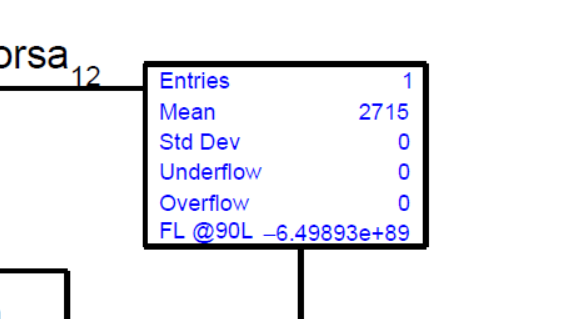Run2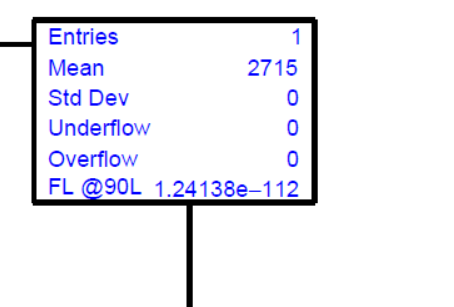Run 3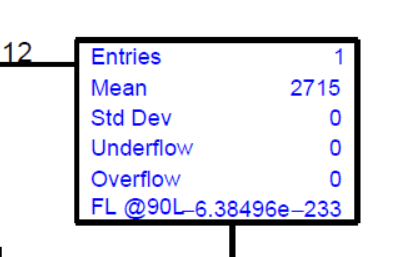Then I tried to calculate the UL of the events instead of the contamination ratio i.e.

`double upperLimit = fc.CalculateUpperLimit(nobs, nback);`

but I get different values each run too…for example:

Run 1

Run 2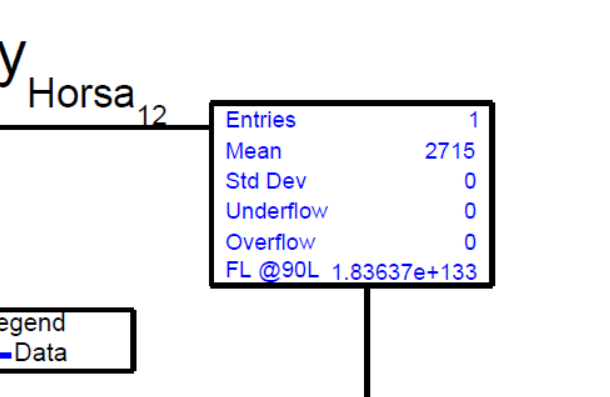Run 3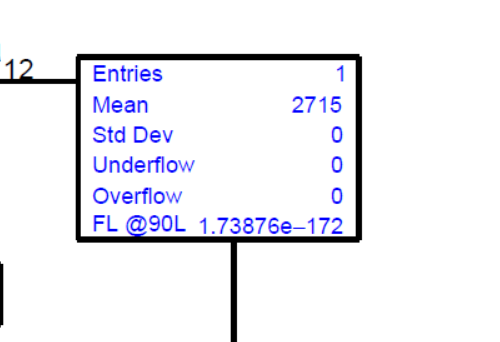Then you see…I get very high or very low different UL values…

HI,
I don’t understand. Do you want the UL on a ratio value (i.e on npassed/ntotal ? )
Then TFeldmanCousins is not the class for you. In TFeldmanCousins nobs must be an integer value and not a ratio.
The correct class in this case is TEfficiency, which computes the confidence interval for the binomial ratio. It includes several methods, including Feldman-Cousins for computing the interval
See https://root.cern.ch/doc/master/classTEfficiency.html

Lorenzo

Hello @moneta I will try to explain you better:

a. I make an energy plot of selected the passing e+/- at the end my detector
i.e. I make this plot

b. I read the entries of this plot to get the selected e+ number…ie. npassed=1
c. I calculate the contamination npassed/ntotal ie. contamination=4.7*10^-5
d. Given that I get a very low selected events, my supervisor said me to apply an UL @90% CL. He said to use the Feldamn Cousin table for that.

You said that in ROOT it’s different the UL on a integer value than on a ratio value…then I will show both the methods to my supervisor.

1. UL using the FL class

If I write on Root Terminal

``````TFeldmanCousins fc;
fc.SetCL(0.90);
double upperLimitFL = fc.CalculateUpperLimit(1, 0.5);
``````

I get the UL 3.86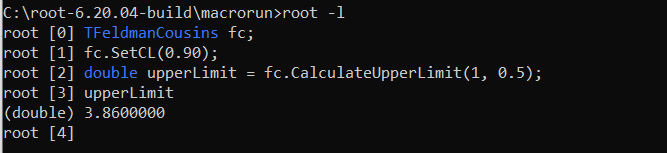but if I write the code

``````TFeldmanCousins fc;
fc.SetCL(0.90);
entries=t->GetEntries();
double upperLimitFL = fc.CalculateUpperLimit(1, 0.5);
.
.
.

statsgenea4sub->AddText(TString::Format("FL @90% CL	= %g", upperLimitFL));
``````

I get different values each time that I run the macro and I get bad values… why?

1. UL using TEfficiency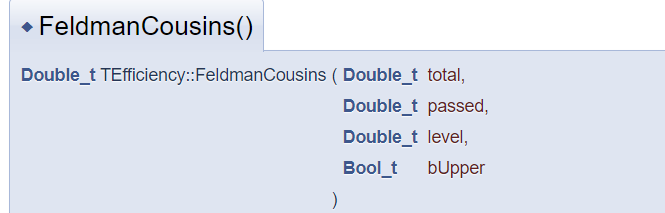Then I did in this way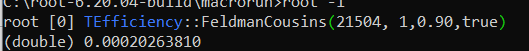is it right?

Hi,

I don’t understand why running the code you get different results. There is maybe a problem in the code. 2 is correct if you want the interval on the rate passed/total.
1 is not for this case

Lorenzo

Thank you @moneta it’s strange that the TFeldmanCousing doesn’t work in the macro because I wrote the same code that I write in the terminal…in the terminal works and in the macro doesn’t…
Anyway thank you!